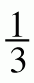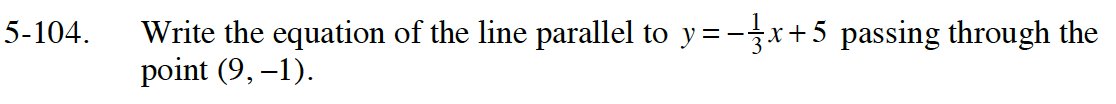Home > INT1 > Chapter Ch5 > Lesson 5.3.2 > Problem5-104

5-104.

Write the equation of the line parallel to y = –x + 5 passing through the point (9, –1). Homework Help ✎Parallel lines have equal slopes. Use y = mx + b where m = slope of the given line.

Use the given point to find the y-intercept.